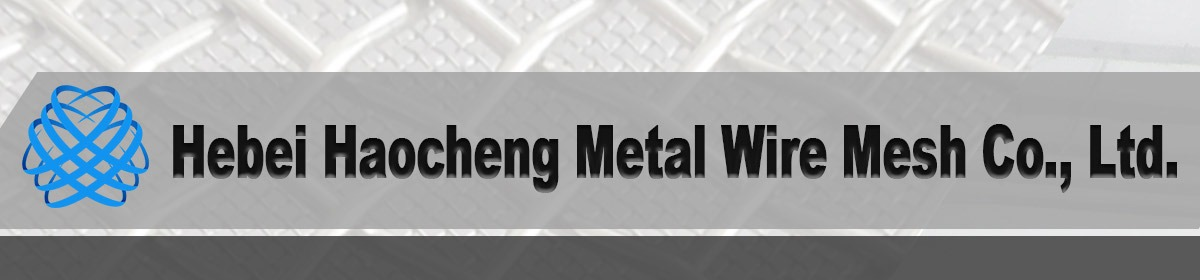• Sourcing Solutions
• Services & Membership
• Help
Orders 0
Favorites 0
Hebei Haocheng Metal Wire Mesh Co., Ltd.
Gold Supplier
Gold Supplier is a premium membership for suppliers on Alibaba.com. Members are provided with comprehensive ways to promote their products, maximizing product exposure and increasing return-on-investment.### Red Copper Wire Mesh

View as: View list View gallery
prev next
Start Order
Place your order online and pay to the designated bank account to get full protection.
Selected Products (0/20)
Click to select products and contact the supplier.
• Min. Order: 1 Square Meter
FOB Price: \$10.80 - \$12.90 / Square Meter
• Min. Order: 1 Square Meter
FOB Price: \$10.80 - \$12.90 / Square Meter
• Min. Order: 1 Square Meter
FOB Price: \$10.80 - \$12.90 / Square Meter
• Min. Order: 1 Square Meter
FOB Price: \$10.80 - \$12.90 / Square Meter
• Min. Order: 1 Square Meter
FOB Price: \$10.80 - \$12.90 / Square Meter
• Min. Order: 1 Kilogram
FOB Price: \$14.00 - \$14.50 / Kilogram
• Min. Order: 1 Square Meter
FOB Price: \$9.80 - \$17.00 / Square Meter
• Min. Order: 1 Square Meter
FOB Price: \$9.80 - \$17.00 / Square Meter
• Min. Order: 1 Square Meter
FOB Price: \$10.80 - \$12.90 / Square Meter
• Min. Order: 1 Square Meter
FOB Price: \$9.80 - \$17.00 / Square Meter
• Min. Order: 1 Square Meter
FOB Price: \$10.80 - \$12.90 / Square Meter
• Min. Order: 1 Square Meter
FOB Price: \$10.80 - \$12.90 / Square Meter
• Min. Order: 1 Square Meter
FOB Price: \$9.80 - \$17.00 / Square Meter
• Min. Order: 1 Square Meter
FOB Price: \$10.80 - \$12.90 / Square Meter
• Min. Order: 1 Square Meter
FOB Price: \$10.80 - \$12.90 / Square Meter
• Min. Order: 1 Square Meter
FOB Price: \$10.80 - \$12.90 / Square Meter
• Min. Order: 1 Square Meter
FOB Price: \$10.80 - \$12.90 / Square Meter
• Min. Order: 1 Square Meter
FOB Price: \$10.80 - \$12.90 / Square Meter
• Min. Order: 1 Square Meter
FOB Price: \$10.80 - \$12.90 / Square Meter
• Min. Order: 1 Square Meter
FOB Price: \$10.80 - \$12.90 / Square Meter
• Min. Order: 1 Square Meter
FOB Price: \$10.80 - \$12.90 / Square Meter
• Min. Order: 1 Square Meter
FOB Price: \$9.80 - \$17.00 / Square Meter
• Min. Order: 1 Square Meter
FOB Price: \$9.80 - \$17.00 / Square Meter
• Min. Order: 1.0 Square Meters
FOB Price: \$5.60 - \$22.90 / Square Meter
• Min. Order: 1.0 Square Meters
FOB Price: \$5.60 - \$22.90 / Square Meter
• Min. Order: 1.0 Square Meters
FOB Price: \$5.60 - \$22.90 / Square Meter
• Min. Order: 1.0 Square Meters
FOB Price: \$5.60 - \$22.90 / Square Meter
• Min. Order: 1.0 Square Meters
FOB Price: \$5.60 - \$22.90 / Square Meter
• Min. Order: 1 Square Meter
FOB Price: \$8.50 - \$19.00 / Square Meter
• Min. Order: 1 Square Meter
FOB Price: \$8.50 - \$19.00 / Square Meter
• Min. Order: 1 Square Meter
FOB Price: \$8.50 - \$19.00 / Square Meter
• Min. Order: 1 Square Meter
FOB Price: \$8.50 - \$19.00 / Square Meter
• Min. Order: 1 Square Meter
FOB Price: \$8.50 - \$19.00 / Square Meter
• Min. Order: 1.0 Square Meters
FOB Price: \$5.60 - \$22.90 / Square Meter
• Min. Order: 1.0 Square Meters
FOB Price: \$5.60 - \$22.90 / Square Meter
• Min. Order: 1.0 Square Meters
FOB Price: \$5.60 - \$22.90 / Square Meter
• Min. Order: 1.0 Square Meters
FOB Price: \$5.60 - \$22.90 / Square Meter
• Min. Order: 1.0 Square Meters
FOB Price: \$5.60 - \$22.90 / Square Meter
• Min. Order: 1.0 Square Meters
FOB Price: \$5.60 - \$22.90 / Square Meter
• Min. Order: 1 Square Meter
FOB Price: \$8.50 - \$19.00 / Square Meter
• Min. Order: 1 Square Meter
FOB Price: \$8.50 - \$19.00 / Square Meter
• Min. Order: 1.0 Square Meters
FOB Price: \$5.60 - \$22.90 / Square Meter
• Min. Order: 1.0 Square Meters
FOB Price: \$5.60 - \$22.90 / Square Meter
• Min. Order: 1.0 Square Meters
FOB Price: \$5.60 - \$22.90 / Square Meter
• Min. Order: 1.0 Square Meters
FOB Price: \$5.60 - \$22.90 / Square Meter
• Min. Order: 1.0 Square Meters
FOB Price: \$5.60 - \$22.90 / Square Meter
• Min. Order: 1 Square Meter
FOB Price: \$8.50 - \$19.00 / Square Meter
• Min. Order: 1 Square Meter
FOB Price: \$8.50 - \$19.00 / Square Meter
• Min. Order: 1 Square Meter
FOB Price: \$8.50 - \$19.00 / Square Meter
• Min. Order: 1 Square Meter
FOB Price: \$8.50 - \$19.00 / Square Meter
prev 1 2 3 4 5 6 next